Computer Science

# Introduction to Bit

## Computers

💻 🖥

Computers do not think. They do not reason.

Computers do EXACTLY what they are told.It’s up to humans to be the intelligent part of the team. But that’s OK - we each play to our strengths.

## The Bit Robot

At the beginning of this course, we’re going to use the Bit robot.

The code for the Bit robot is in the file `byubit.py`.

You’ll need a copy of this file on your computer to write scripts that use Bit.

We’ll provide instructions in the lab for how to download the file, but the gist of it is:

``````conda activate cs110
pip install byubit``````

Bit lives in a grid world, like a chessboard.

He can do four actions: `move`, `left`, `right`, and `paint`.

Let’s see what that means.

``````from byubit import Bit
bit = Bit.new_world(5,3)
bit.draw()``````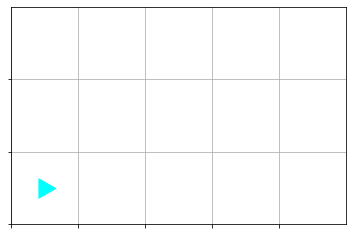``````bit.move()
bit.move()
bit.draw()``````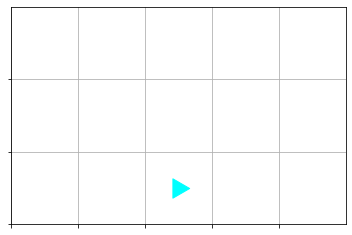``````bit.left()
bit.move()
bit.move()
bit.paint("green")
bit.draw()``````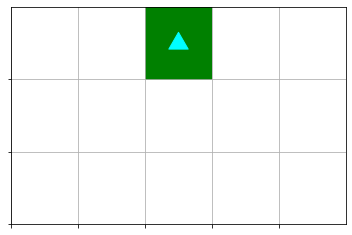### Let’s try it together!

If you already have `byubit` installed, open a text editor and type in the following:

``````from byubit import Bit
bit = Bit.new_world(5,3)

bit.draw()

bit.move()
bit.move()
bit.draw()``````
``````bit.left()
bit.move()
bit.move()
bit.paint("green")
bit.draw()
``````

Save your file as `try_bit.py`.

``python try_bit.py``

What did you get?

## What does all this mean?

``from byubit import Bit``

We’ll talk about this more later in the course. For now, it is accurate and useful to understand that this line means we are using code someone else wrote.

But you’ll probably notice that `byubit` matches the name of the package in `pip install byubit`

``bit = Bit.new_world(5,3)``

First, notice that `Bit` and `bit` have different capitalization (a.k.a. case or casing). In python, and most other programming languages, the case of the letters matter. So, `Bit` and `bit` mean different things.

`Bit` is that thing we `import`ed from `byubit` (whatever that means—don’t worry, we’ll cover that later).

Next to `Bit` is `new_world` and the numbers `5`, and `3`. You’ll notice that the bit world we looked at had 5 squares left-to-right, and 3 squares top-to-bottom.

`bit` is the thing that shows up over and over in our script.

`bit` is an example of a variable.

A variable is a name we give to a piece of information in a program.

It’s like “it” in the game of tag: “Sally is ‘it’—run!”

We use `=` to give a variable a value. Whatever `Bit.new_world(5,5)` means, `bit` now represents that.

If I were writing a program that simulated a game of tag, I might start with:

``it = "Gordon"``

If, after Gordon tags Daniel, I might have a line:

``it = "Daniel"``
``````bit.move()
bit.left()
bit.paint("green")``````

When `bit` `move`s, `left`s, `right`s, or `paint`s, the little cyan triangle moves, turns, and paints.

(By the way, `bit` can also paint `"blue"`, and `"red"`).

The `move`, `left`, and `paint` words refer to functions.

Functions are code written somewhere else that we want to use.

When we use a function, we say that we invoke or call a function.

Sometimes we can give a function information to use. We call this information arguments or args for short.

For example, `bit.paint` takes one argument: a word indicating the color we want painted.

`bit.move` or `bit.left` take no arguments.

We’ll talk more about functions later.

We’ll talk more about the `.`, `=`, `"`, `()`, etc. later on. These are part of the python syntax.

Syntax refers to the specific way our instructions to the computer must be written (computers are very particular about this kind of thing).

## Abstractions

### What is a car?

🚗 🚙 🏎 🚃 🚠

Abstractions have meaning. They communicate a set of qualities but leave out the details.

CAR - has wheels, carries people, needs fuel

Within the appropriate scope, we can reason and communicate in abstractions.

I don’t have to tell you that I drove to work today in a 2008 Toyota Yaris. I can say “I came in my car” and that’s good enough.

Abstractions protect our brains from information overload. We can worry about only the relevant details.

Programming is all about using and creating abstractions.

`bit` is an abstraction.

You don’t need to understand the nitty-gritty details of `bit`. You don’t even need to understand how `bit`works. It’s enough to simply understand that `bit.move()` will move the Bit, `bit.paint("green")` will make the square green, `bit.draw()` will show you a picture of Bit’s world, etc.

## Syntax

Remember, computers are very particular about how things are written.

It’s like my kids correcting each other’s pronunciation (Michaela: MUH-kay-luh 🤦🏻‍♂️)

Computers are not smart. They have to be told exactly what to do, and part of that exactness is stating your desires in a format, or syntax, the computer understands perfectly.

Humans, however, tend to be significantly more flexible than computers in how things are communicated.

To-MAY-to, to-MAH-to, no big deal.

This inevitably leads to moments of conflict.

👩🏻‍🦰 vs 💻

When we humans fail to follow the computer’s expected syntax perfectly, the computer will be quick to say so.

This is not an indication that you are bad programmer. I get these ALL. THE. TIME.

It just means you are a human and your companion is a computer.

``bit.move(]``

File “/tmp/ipykernel_586/2122551420.py”, line 1 bit.move(] ^ SyntaxError: closing parenthesis ’]’ does not match opening parenthesis ’(’

Sometimes the computer is good at telling us what the problem is.

Other times…not so much.

``bit=Bit-new_world()``
``````    ---------------------------------------------------------------------------

NameError                                 Traceback (most recent call last)

/tmp/ipykernel_586/4273431528.py in <module>
----> 1 bit=Bit-new_world()

NameError: name 'new_world' is not defined``````
``bit=Bit.new_world(``
``````      File "/tmp/ipykernel_586/1497934548.py", line 1
bit=Bit.new_world(
^
SyntaxError: unexpected EOF while parsing``````
``bit=Bit.new_world(7)``
``````    ---------------------------------------------------------------------------

TypeError                                 Traceback (most recent call last)

/tmp/ipykernel_586/950519080.py in <module>
----> 1 bit=Bit.new_world(7)

TypeError: new_world() missing 1 required positional argument: 'size_y'``````
``bit.move(7)``
``````    ---------------------------------------------------------------------------

TypeError                                 Traceback (most recent call last)

/tmp/ipykernel_586/2203445208.py in <module>
----> 1 bit.move(7)

TypeError: move() takes 1 positional argument but 2 were given``````
``bit.paint("blue)``
``````      File "/tmp/ipykernel_586/3689294578.py", line 1
bit.paint("blue)
^
SyntaxError: EOL while scanning string literal``````
``bit.paint(red)``
``````    ---------------------------------------------------------------------------

NameError                                 Traceback (most recent call last)

/tmp/ipykernel_586/227196410.py in <module>
----> 1 bit.paint(red)

NameError: name 'red' is not defined``````
``bit.paint(blue")``
``````      File "/tmp/ipykernel_586/1056244548.py", line 1
bit.paint(blue")
^
SyntaxError: EOL while scanning string literal``````
``bit.turn_left()``
``````    ---------------------------------------------------------------------------

AttributeError                            Traceback (most recent call last)

/tmp/ipykernel_586/2647963323.py in <module>
----> 1 bit.turn_left()

AttributeError: 'Bit' object has no attribute 'turn_left'``````
``bit . left()``

What!? That…worked?

🤨

We’ll talk more about the sytax of python as we go, and you’ll see more and more examples of python, and you’ll get a feel for what is valid syntax and what isn’t.

You’ll learn the difference between to-MAY-to 🍅 ✅

to-MAH-to 🍅 👍🏻

and to-MUU-to 🐄 🤪

### Other kinds of errors

Not all errors are syntax errors. Sometimes you gave your instructions in the correct syntax, but your instructions just don’t make sense.

It’s like the statement:

Let’s eat, Grandpa

If you leave out the comma you get:

Let’s eat Grandpa

The (english) syntax is still correct, but the message communicated is probably not what you intended.

These kinds of errors can manifest in various ways.

``6 / 2  # this works fine``
``    3.0``
``7 / "a cow"``
``````    ---------------------------------------------------------------------------

TypeError                                 Traceback (most recent call last)

/tmp/ipykernel_586/4290478088.py in <module>
----> 1 7 / "a cow"

TypeError: unsupported operand type(s) for /: 'int' and 'str'``````

Sometimes the result is more subtle.

``````from byubit import Bit
bit = Bit.new_world(3,3)
bit.draw()
bit.move
bit.draw()``````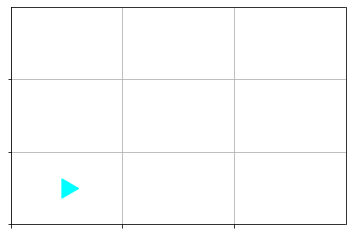Do you see the problem?

Not all errors are caused by incorrect syntax.

Sometimes errors are cause by contraints in your program.

For example:

``````bit = Bit.new_world(2,1)
bit.draw()``````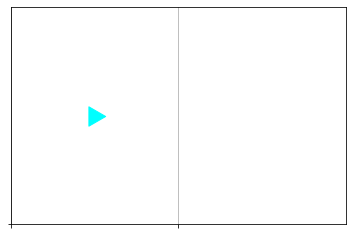``````bit.move()
bit.draw()``````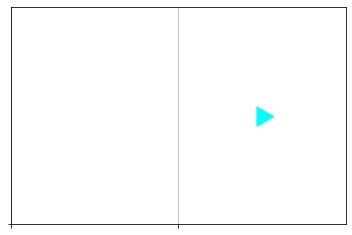``````bit.move()  # is this going to work?
bit.draw()``````
``````    ---------------------------------------------------------------------------

MoveOutOfBoundsException                  Traceback (most recent call last)

/tmp/ipykernel_586/21756017.py in <module>
----> 1 bit.move()  # is this going to work?
2 bit.draw()

/opt/conda/lib/python3.9/site-packages/byubit.py in move(self)
178         next_pos = self._get_next_pos()
179         if not self._pos_in_bounds(next_pos):
--> 180             raise MoveOutOfBoundsException(f"Bit tried to move to {next_pos}, but that is out of bounds")
181         elif self._get_color_at(next_pos) == BLACK:
182             raise MoveBlockedByBlackException(next_pos)

MoveOutOfBoundsException: Bit tried to move to [2 0], but that is out of bounds``````

These kinds of errors are called runtime errors because they happen while your code is running, and not just at the beginning while the computer is reading your instructions.

Remember, we ALL get errors while writing code. It’s not a sign that you’re a bad programmer, it just means you need to fix something to make the computer happy.

## A Comment

The python syntax allows the programmer to include comments in the code.

The computer will completely ignore everything following `#` to the end of the line.

It’s as if the stuff isn’t even there.

🥷🏿

``````# Load a new Bit world
bit = Bit.new_world(5,3)

# Move bit twice, turn left, move again, then paint
bit.move()
bit.move()
bit.left()
bit.move()
# bit.paint("green")
bit.paint("blue")``````

## More Bit

Let’s play some more with Bit. This is for you to try. 🙂

``````bit = Bit.new_world(2, 3)
bit.draw()``````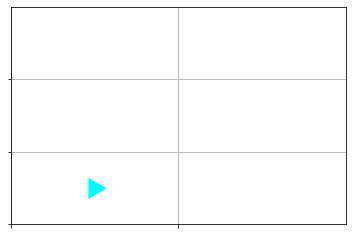Bit is in the bottom left corner, facing right.

Now make the world look like this: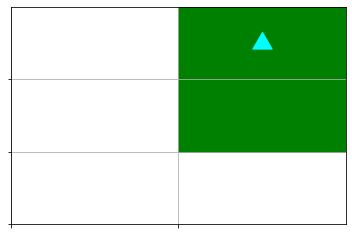Don’t worry if you make some mistakes at first. You’ll get good at this soon enough.

``````from byubit import Bit
bit = Bit.new_world(2,3)
bit.move()
bit.left()
bit.move()
bit.paint("green")
bit.move()
bit.paint("green")
bit.draw()``````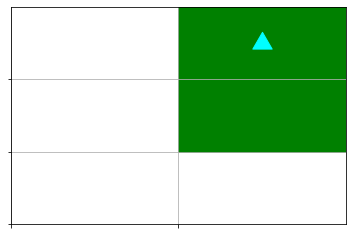Did you get it?

👨🏻‍💻🧑🏾‍💻👩🏼‍💻 ✅

👨🏼‍🎓 👩🏽‍🎓

### What happens when you color the same square twice?

``````from byubit import Bit
bit = Bit.new_world(3,3)
bit.paint("green")
bit.paint("blue")
bit.draw()``````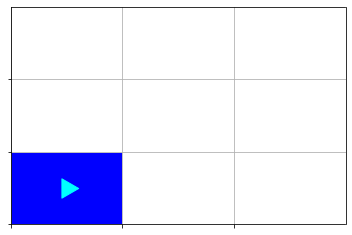``````from byubit import Bit
bit = Bit.new_world(3,3)
bit.paint("green")
# Add an extra bit.draw() to see what happens in the middle
bit.draw()
bit.paint("blue")
bit.draw()``````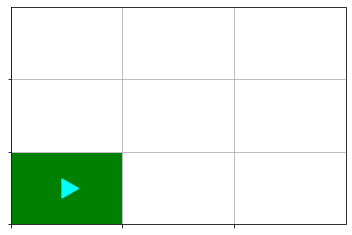The bit simply paints over the old color.

## Share the Bit

You can share your Bit worlds.

``bit.save("my-bit.txt")``

Bit saved to my-bit.txt

``````bit = Bit.load("my-bit.txt")
bit.draw()``````## Compare the Bit

You can compare your Bit world to another.

For example: for many of the exercises we give you, you’ll be given an expected world to compare to.

``````bit = Bit.new_world(5,3)
bit.move()
bit.move()
bit.paint("green")``````
``````expected_bit = Bit.load("expected.txt")
bit.compare(expected_bit)``````
``    Location of Bit does not match: (2, 0) vs (2, 1)``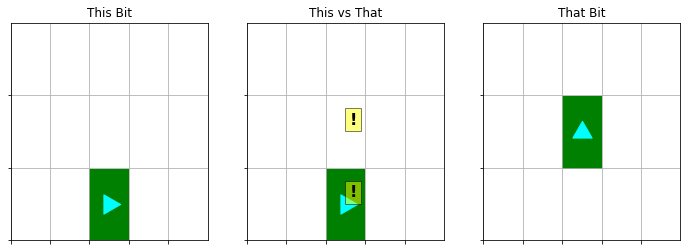``    False``

## Go Green

Now, let’s say I want you to make the following:To make all that green, you’d need to repeat lots of

``````bit.move()
bit.paint("green")``````

over and over.

You’d also want a way of knowing when to stop.

Students frantically start to copy-paste code over and over

😱

There is a better way!

``````bit = Bit.new_world(10,3)
while bit.front_clear():
bit.move()
bit.paint("green")
bit.draw()``````That was it!?

🤨 🤔 😅

Let’s break it down.

``````bit = Bit.new_world(10,3)
while bit.front_clear():
bit.move()
bit.paint("green")
bit.draw()``````

`while bit.front_clear()` - what does this mean?

Notice the indentation of `bit.move()` and `bit.paint("green")`. This is important python syntax.

Notice the lack of indentation for `bit.draw()`. What would happen if you indented it like `bit.move()`?

### Return values

Sometimes code simply does something and that’s the end of it. The code has nothing to give you when it is done.

``bit.move()``

But sometimes code has a return value: that piece of code has information to give back when it is done.

``bit.front_clear()``

`bit.front_clear()` returns a boolean, which is either `True` or `False`.

``````bit = Bit.new_world(2,1)
bit.draw()
print(bit.front_clear())

bit.move()
bit.draw()
print(bit.front_clear())````````    True````    False``

The `while` expression requires a boolean, a `True` or `False`. `bit.front_clear()` can give it one.

### `while` Loop Syntax

``````while <expression>:
Indended statements that happen while <expression> is True``````

### `while` Loop Operation

• Check `<expression>`
• If it is `True`, run the indented code following the `while` statement
• If it is `False`, skip the indented code
``````while bit.front_clear():
bit.move()``````

### `while` Loop Observations

• The code before and after a `while` block are run
• You can have many lines of code in the block body
• The body of the `while` loop can run many times (or no times!)

## Generality

One piece of code, but many cases served

``````bit = Bit.new_world(5,3)
while bit.front_clear():
bit.move()
bit.paint("green")
bit.draw()````````````bit = Bit.new_world(15,5)
while bit.front_clear():
bit.move()
bit.paint("green")
bit.draw()````````````bit = Bit.new_world(50,20)
while bit.front_clear():
bit.move()
bit.paint("green")
bit.draw()``````### `while` Loop Power!

These things are awesome.

“Do this same thing over and over until I tell you to stop.”

There several kinds of loops. Today we talked about `while`.

## Summary

• Computers and humans play to their strengths - we need to speak their language.
• Bit is a tool - a “library” to help you learn the first basics of programming.
• Abstractions are things we can work with even if we don’t know how they work inside.
• `while` loops are an abstraction that let us repeat instructions to the computer under specified conditions